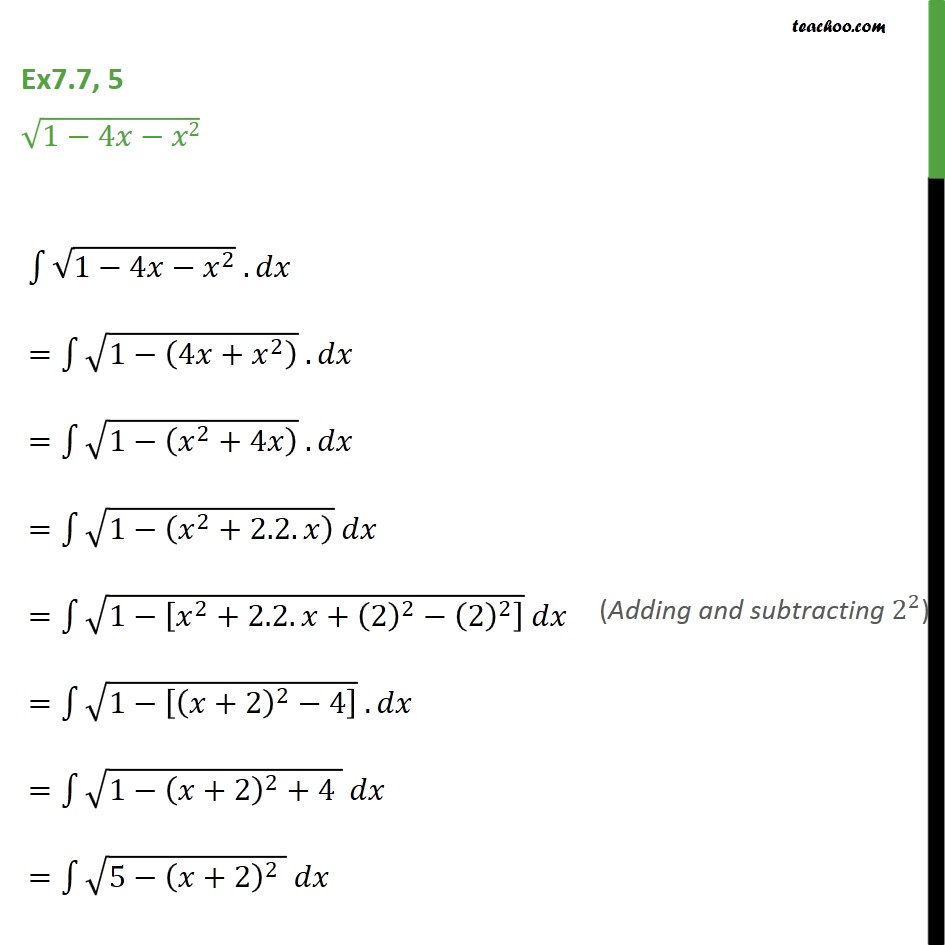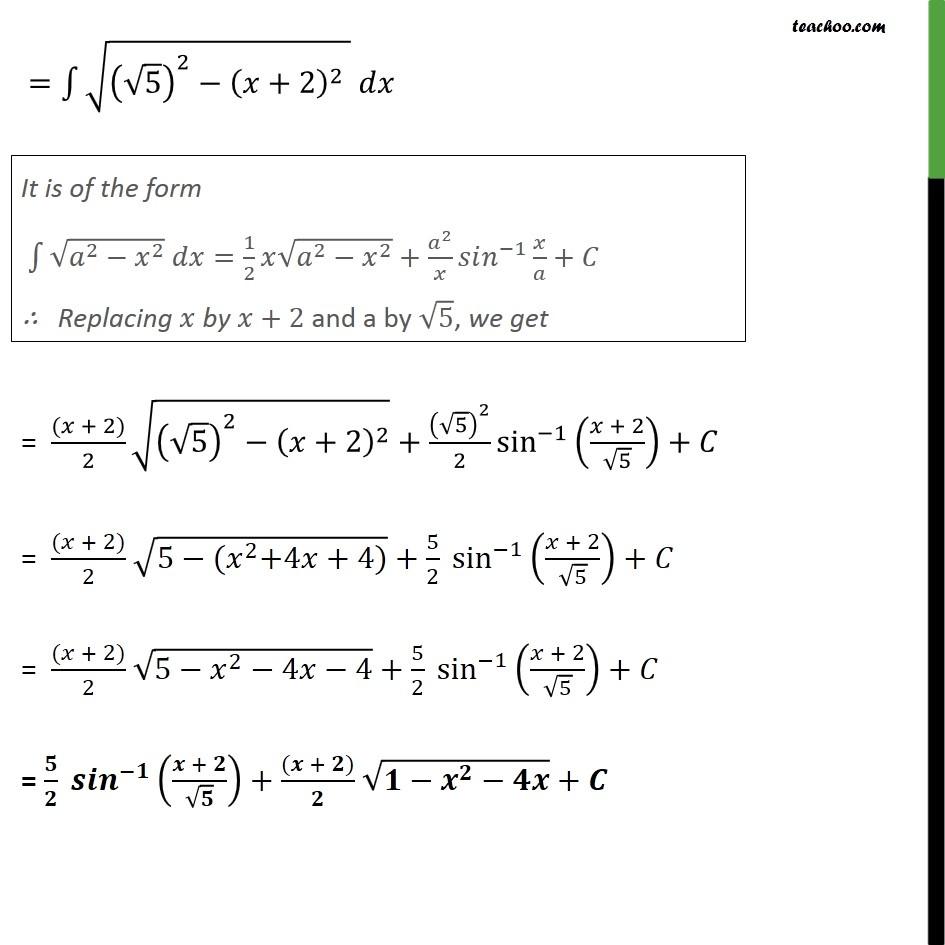Ex 7.7

Chapter 7 Class 12 Integrals
Serial order wiseLearn in your speed, with individual attention - Teachoo Maths 1-on-1 Class

### Transcript

Ex7.7, 5 (1 4 2) 1 (1 4 ^2 ) . = 1 (1 (4 + ^2 ) ) . = 1 (1 ( ^2+4 ) ) . = 1 (1 ( ^2+2.2. ) ) = 1 (1 [ ^2+2.2. +(2)^2 (2)^2 ] ) = 1 (1 [( +2)^2 4] ) . = 1 (1 ( +2)^2+4 ) = 1 (5 ( +2)^2 ) = 1 (( 5)^2 ( +2)^2 ) = (( + 2))/2 (( 5)^2 ( +2)^2 )+( 5)^2/2 sin^( 1) (( + 2)/ 5)+ = (( + 2))/2 (5 ( ^2+4 +4))+ 5/2 sin ^( 1) (( + 2)/ 5)+ = (( + 2))/2 (5 ^2 4 4)+5/2 sin ^( 1) (( + 2)/ 5)+ = / ^( ) (( + )/ )+(( + ))/ ( ^ )+